## Example Questions

### Example Question #291 : Algebra

Susan has one gallon of 25% acid solution, and she needs 75% acid solution for her experiment. How much pure acid should she add?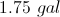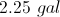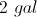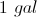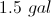Explanation:

Pure water is 0% and pure acid is 100%.

The general formula to use is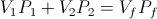, whereis the volume andis the percent.

Our known values are listed below.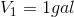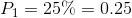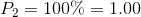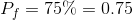We will call our unknown added acid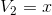.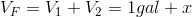So the equation to solve becomes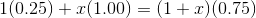.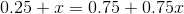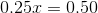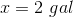Sally sells hair accessories. Her monthly fixed costs are $150. It costs her$5 to make each barrette, and she sells them for $10 each. How many barrettes must Sally sell in order to break even with her costs? Possible Answers: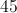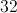Correct answer:Explanation: The break-even point occurs when the revenues are the same as the costs. Letbe the number of barrettes sold. Revenue is equal to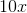. Cost is equal to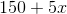. The equation to solve becomes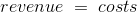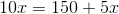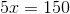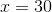Solving shows that 30 barrettes must be sold to break-even. ### Example Question #21 : How To Find The Solution To An Equation Sally sells hair accessories. Her monthly fixed costs are$150. It costs her $5 to make each barrette, and she sells them for$10 each.

If Sally wants to make a profit of $250, how many barrettes must she sell? Possible Answers: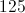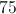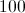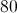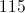Correct answer:Explanation: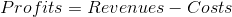Letbe equal to the number of barrettes sold. Revenue will be equal to. Costs will be equal to. The equation to solve becomes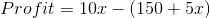.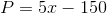To reach the profit goal of$250, we need to calculate the value of.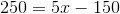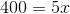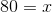### Example Question #1791 : Act Math

What are the values for x that satisfy the equation (x-d)(x+c)=0?

d and c

dc

-dc

d and -c

–d and c

d and -c

Explanation:

Set each component of this equation equal to zero and solve for d and c.

(x-d)(x+c)=0

x-d=0

d=0

x+c=0

x=-c

### Example Question #21 : How To Find The Solution To An Equation

If A + B = C and B – C = 3, what does A equal?

3

3

9

6

9

3

Explanation:

This question can be solved using substitution. There are several ways to subsitute, but one possible method is to substitue (A+B) for C in  the equation B-C=3: B-(A+B)=3. This simplifies to (B-A-B=3) (don't forget to distribute the negative!). Since (B-B)=0, -A must equal 3 (-A=3). If we divide both sides by -1, A=-3. If you got an answer of positive 3, you may have forgotten to divide both sides by -1.

### Example Question #22 : How To Find The Solution To An Equation

The population of a bird species is modeled by the following equation: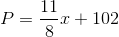,

whererepresents the number of years from the present. How many years will it take the population to reach 130 birds (rounded to the nearest tenth)?

280.8

12.2

38.5

23.0

20.4

20.4

Explanation:

Plugging in 130 for P, the equation becomes 130 = (11/8)x + 102.  Solving for x, we obtain x = 20.4 years.

### Example Question #1793 : Act Math

What is the value of c when 3c + 4 = 2c  7?

0

11

11

1

No Solution

11

Explanation:

First put the variables on one side by subtracting 2c on both sides to get c + 4 = –7. Then subtract the 4 on both sides to arrive at your answer, c = 11.

### Example Question #1792 : Act Math

If y=0.35(300 – 2y), what is the value of y to the nearest tenth?

62.3

51.2

61.8

65.0

61.8

Explanation:

Distributing the 0.35 to both the 300 and 2y leaves y=105 0.7y

Adding 0.7y to both sides and dividing by 1.7 gives 61.8.

### Example Question #1794 : Act Math

Which of the lines below is not parallel to 4x – 3y = 17 ?

–y= –(4/3)x – (17/3)

8x + 6y = 34

8x  6y = 34

2x  1.5y = 8.5

8x + 6y = 34

Explanation:

For a line to be parallel with another, the slopes must be equal. All of the equations have a slope of 4/3 except 8x +6y=34.

### Example Question #21 : Linear / Rational / Variable Equations

If the expression x4 + 3cx  2 is equal to 5 when x = 1, what is the value of c?

3

1

2

2